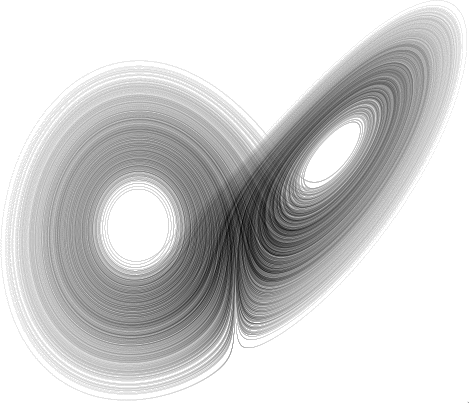The CAPD library has been used in several articles in which chaotic dynamics, bifurcations, heteroclinic/homoclinic solutions and periodic orbits were studied.

 Chaotic dynamics for various ODE's By chaotic dynamics we often understand semi-conjugacy of the map restricted to an invariant set to the shift map on some number of symbols. In this situation we will say that the map has symbolic dynamics. For this purpose some methods were developed. Very pioneer works with an application to an ODE are [1,2,3], where the authors verified that a Poincare map for the well known Lorenz system x' = σ(y-x),   y' = x(ρ-z)-y,   z' = xy-βz for parameter values (σ,ρ,β)∊{(10,28,8/3),(10,60,8/3),(10,54,45)} has symbolic dynamics. They used a method based on discrete version of the Conley index. Later, Piotr Zgliczynski  proposed a method based on the Brouwer degree theory and called the method of covering relations. The method has been proved to be very efficient and it has various applications to: the Henon map and the Rossler system x' = -y-z,   y' = x+by,   z' = b+z(x-a) for "classical" parameter values b=0.2, a=5.7  the Lorenz system for "classical" parameter values (σ,ρ,β)=(10,28,8/3)  the Michelson system x''' + x' + 0.5x2 = c2 for parameter c=1 . In  the semi-conjugacy to the full shift on four symbols for an interval of parameters c∊[0.8285,0.861] is proved. the hyperchaotic Rossler system x' = -y-w,   y' = x+ay+z,   z' = dz+cw,   w' = xw+b for the "classical" parameters (a,b,c,d)=(0.25,3,-0.5,0.05) . In this case the Poincare map exhibits an attractor with two expanding directions. the Planar Restricted Circular Three Body Problem [9,10] for the masses and energy level corresponding to the Oterma comet in the Sun-Jupiter system. In each of these results except  the authors used rigorous C0-solver from the CAPD library to integrate the system and to obtain a verified enclosure for Poincare maps. References: K. Mischaikow, M. Mrozek, Chaos in Lorenz equations: a computer assisted proof, Bull. Amer. Math. Soc. (N.S.), 33(1995), 66-72. K. Mischaikow, M. Mrozek, Chaos in the Lorenz equations: a computer assisted proof. Part II: details, Mathematics of Computation, 67 (1998), 1023-1046. K. Mischaikow, M. Mrozek, A. Szymczak, Chaos in the Lorenz equations: a computer assisted proof. Part III: the classical parameter values, J. Diff. Equ. 169(2001), 17-56. P. Zgliczynski, Computer assisted proof of chaos in the Henon map and in the Rossler equations, Nonlinearity 10, 243-252 (1997). Z. Galias, P. Zgliczynski, Computer assisted proof of chaos in the Lorenz system, Physica D, 115, 165-188 (1998). D. Wilczak, Chaos in the Kuramoto-Sivashinsky equations - a computer assisted proof, Journal of Differential Equations, Vol.194, 433-459 (2003). D. Wilczak, The existence of Shilnikov homoclinic orbits in the Michelson system: a computer assisted proof, Foundations of Computational Mathematics, Vol.6, No.4, 495-535 (2006). D. Wilczak, Abundance of heteroclinic and homoclinic orbits for the hyperchaotic Rossler system, Discrete and Continuous Dynamical Systems - Series B, Vol. 11, No. 4, 1039-1055 (2009). D. Wilczak, P. Zgliczynski, Heteroclinic Connections between Periodic Orbits in Planar Circular Restricted Three Body Problem - A Computer Assisted Proof, Communications in Mathematical Physics, Vol.234, No.1, 37-75 (2003). D. Wilczak, P. Zgliczynski, Heteroclinic Connections between Periodic Orbits in Planar Circular Restricted Three Body Problem - part II, Communications in Mathematical Physics, Vol. 259, No.3, 561-576 (2005). Applet written by Marian Mrozek, which shows dynamics of the Lorenz equations.Attractors of the Lorenz and 3D Rossler equations.